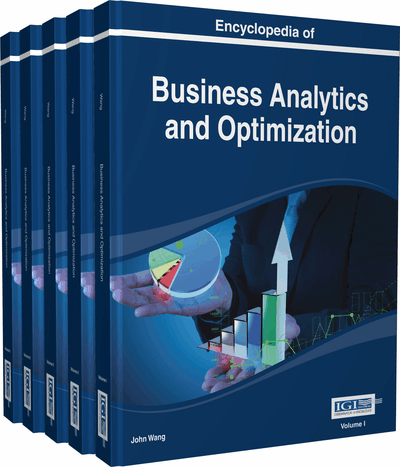# ROC Analysis in Business Decision-makings

Nan Hu (University of Utah, USA) and Xiao-Hua Zhou (University of Washington, USA)
DOI: 10.4018/978-1-4666-5202-6.ch189

Top

## Main Focus Of The Chapter

We first define accuracy parameters of binary classification tools, and then extend the evaluation method to test modalities with continuous or discrete ordinal values. By applying accuracy parameters and ROC analysis, business analysts can easily examine the expected downstream harms and benefits of positive and negative test results based on these test modalities, and directly link the classification accuracy to important decision-makings (Cornell, Mulrow, & Localio, 2008).

## Key Terms in this Chapter

Specificity: the probability that a diagnostic test can correctly specify a non-case.

Diagnostics Test: a quantitative test modality that is used to discriminate cases of interest from non-cases (controls).

False Positive Rate: the probability that a diagnostic test incorrectly classify a control as a case.

AUC (C-Statistic): the area under an ROC curve that summarize the overall probability for correct classification.

ROC Curve: a curve that plots a diagnostic test’s sensitivity versus its false positive rate across all possible threshold values for defining positivity.

Sensitivity: the probability that a diagnostic test can correctly identify a true case.

Gold Standard: a standard that can specify the true status being evaluated without error (a.k.a., reference standard).

False Negative Rate: the probability that a diagnostic test classify incorrectly a case as a control.

## Complete Chapter List

Search this Book:
Reset